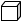# Evaluate Cubes

What does it mean to be cubed? Start with visualizing a cube. We know that a cube has 3 dimensions: length, width and height and all three are the same length. To find the volume of a cube we multiply length times width times height. If we let x represent the side length we can write the formula V = x3 which means the volume is x •x •x . Cubed means to multiply a number by itself 3 times.

x3=x •x •x

Let's practice with numbers.

Example 1: 43 = 4 •4 •4 = 64

Let's practice evaluating with variables.

Example 2: find x3 if x = 5.

Substitute (5) in for x.
x3 = (5)3

Expand
x3 = 5 •5 •5 = 125

Therefore
x3 = 125

Let's look at a common mistake.

Remember that x3 = x •x •x so what does - x3 represent? It means (-1) •x •x •x

Example 3: find - x3 when x = 1

Substitute (1) in for x.
-x3 = - (1)x3

Expand
-x3= -1 •1 •1 •1 = -1

Therefore
-x3 = -1

Challenge: find 3 x2 y3 when x = 4 and y = 3

Substitute (4) for x and (3) for y
3 x2 y3 = 3 • (4)2 • (3)3

Expand
3 x2 y3 = 3 •(4) •(4) •(3) •(3) •(3)

3 x2 y3 = 1296

Let's sum this up. Remember that to be "cubed" means to multiply a number times itself three times.

 Related Links: Math algebra Evaluate Exponents

To link to this Evaluate Cubes page, copy the following code to your site: